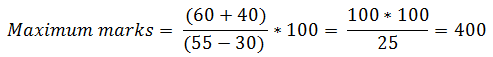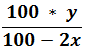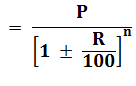New Students Offer - Use Code HELLO

# Tricks to solve Percentage Problems - PDF

Percentage is a fraction whose denominator is always 100. x percentage is represented by x%.

### Calculation of Percentage

If we have to find y% of x, then### 1. To express x% as a fraction :

We know
x% = x/100
Thus 10% = 10/100 (means 10 parts out of 100 parts)
= 1/10 (means 1 part out of 10 parts)

### 2. To express x/y as a percentage :

We know that x/y  = (x/y× 100 )
Thus 1/4 = ( 1/4 ×100 )% = 25%
and 0.8 = ( 8/10 ×100 )% = 80%

### 3. To increase a number by a given percentage(x%):

Multiply the number by the following factor### 4. To decrease a number by a given percentage(x%):

Multiply the number by the following factor

### 6. To find the % decrease of a number:## Some Observation

### #1

If 20% candidate failed in an exam then observations are
• 80% represent passed in exam
• 100% represent total appeared in exam
• (80%-20%) = 60% represent difference between passed and failed candidate in exam

### #2

If a number is increased by 25% then observations are
• 100% represent the old number
• 125% represent the new number.

### #3

Remember that Base in the given sentence (Question) is always 100%
Eg. Income of Ram is increased by 20%
In this sentence
100% - represent the income of Ram
20% - represent increment
120% - represent new income of Ram.

#### Remember it :

1 = 100%
1/2 = 50%
1/3 = 33 1/3%
1/4 = 25%
1/5 = 20%
1/6 = 162/3%
1/7 = 142/7%
1/8 = 121/2%
1/9 = 111/3%
1/10 = 10%
1/11 = 91/11%
1/10 = 81/3%
1/13% = 79/13%
25% = 1/4
6.25% = 1/16
125% = 5/4
150% = 3/2
200% = 2
350% = 7/2

### #4

If of A is equal to y% of B then -
Example: - If 10% of A is equal to 12% of B, then 15% of A is equal to what percent of B?

### #5

If A is more than B,
Example: - If income of Ravi is 20% more than that of Ram, then the income of Ram is how much percent less than that of Ravi?

### #6

If the passing marks in an examination is P%. If a candidate scores S marks and fails by F marks then–
Example: - Pankaj Sharma has to score 40% marks to get through. If he gets 40 marks and fails by 40, then find the total marks set for the examination?

### #7

If a candidate scores marks and fails by a marks while an another candidate scores y% marks and gets b marks more than minimum passing marks, then –

Example: - Raj scores 30% and fails by 60 marks, while Rohan who scores 55% marks, gets 40 marks more than the minimum required marks to pass the examination. Find the maximum marks for the examination?### #8

If due to decrement in the price of an item, a person can buy Kg more in y rupees, then actual price of that item -
Example: - Ram can buy 5 Kg more sugar in rupees 100 as the price of sugar has decreased by 10%. Find the actual price of the sugar?### #9

If in an election, a candidate got of total votes cast and still lose by y votes, the total number of votes cast –Example: - In an election contested by two candidates, one candidate got 40% of total votes and still lost by 500 votes, find the total number of votes casted?### #10

If the population of a town is P and it increases or decreases at the rate of R% per annum then –
I. Population after ‘n’ years :II. Population ‘n’ years ago :Example: - The population of a town is 352800. If it increases at the rate of 5% per annum, then what will be its population after 2 years and 2 years ago?

### #11

If the value of a number is first increased by and again decreased by the net effect is always decreased by x2/100%
Example: -The salary of a worker is first increased by 5% and then it is decreased by 5%. What is the change in his salary?

## Examples

### #1

Q. If the difference between 62% of a number and 3/5th of that number is 36. what is the number ?
Sol:
Let the number be x.
Then x × 62% - x × 3/5 = 36
x ×62% -x V 60% = 36 (60% = 3/5)
x ×2% = 36
x ×2/100 =36
x = 36 ×100/2 = 1800

### #2

Q. 40% of Ram's income Rs. 1200 Then Find
1. 75% of Ram's income ?
2. 1/4 part of Ram's income ?
3. 1/3 part of Ram's income ?
Sol :
(1)
40% = 1200 Rs.
75% = 1200/40 ×75 = 2250 Rs.

#### Trick : 1200 / 40  × 75 = Rs. 2250/-

(2)
40% of income = Rs. 1200
Then 1/4 part (i.e. 25% ) of Ram's
income = 1200/40 ×25
= Rs. 750/- Ans

(3)
40% of Ram's income
= Rs. 1200
i.e. 2/5 part of Ram's income
= Rs. 1200
Then total income of Ram
= Rs. 1200 ×5/2
1/3 part of Ram's income
= Rs. 1200  × 5/2  × 1/3
= Rs. 1000 Ans.

#### Trick :

1200/2/5  × 1/3
= 1200/2 × 5/3 = 1000